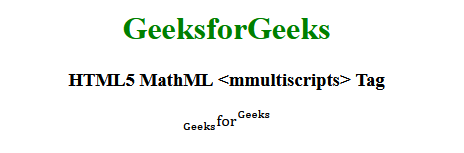Related Articles
HTML5 | MathML <mmultiscripts> Tag
• Last Updated : 08 Nov, 2019

The MathML <mmultiscripts> tag in HTML5 is used to create multi-dimensional matrices. The degree depends on the conditionality of a representative array. As a number/digit is a 0-dimensional array. 1st order Tensor and 2nd order tensor as a squire matrices will represent a 1-dimensional array.

Syntax:

` <mmultiscripts> child elements </mmultiscripts>`

Attributes: This tag accepts below mentioned attributes:

• class|id|style: This attribute holds the styles of the child elements.
• href: This attribute holds any hyperlink to a specified URL.
• mathbackground: This attribute holds the value of the math expressions background color.
• mathcolor: This attribute holds the color of the math expressions.
• subscriptshift: This attribute defines the minimum space by which to shift the subscript below the baseline of the expression.
• superscriptshift: This attribute defines the minimum space by which to shift the subscript above the baseline of the expression

Below example illustrates the concept of HTML5 MathML <mmultiscripts> tag:

Example:

 ```<``html``>``  ` `<``head``>``    ``<``title``>HTML5 MathML mmultiscripts tag````  ` `<``body``>``    ``<``center``>``        ``<``h1` `style``=``"color:green"``>GeeksforGeeks``         ` `        ``<``h3``>HTML5 MathML <``mmultiscripts``> Tag``         ` `        ``<``math``>``            ``<``mmultiscripts``>``                ` `                ````                ``<``mi``>for``  ` `                ````                ``<``none` `/>``  ` `                ````                ``<``mi``>Geeks``  ` `                ``<``mprescripts` `/>``  ` `                ````                ``<``mi``>Geeks``  ` `                ````                ``<``none` `/>``  ` `            ````        ````    ``````  ` ``

Output:Supported Browsers: The browsers are supported by HTML5 MathML <mmultiscripts> tag are listed below:

• Firefox

My Personal Notes arrow_drop_up